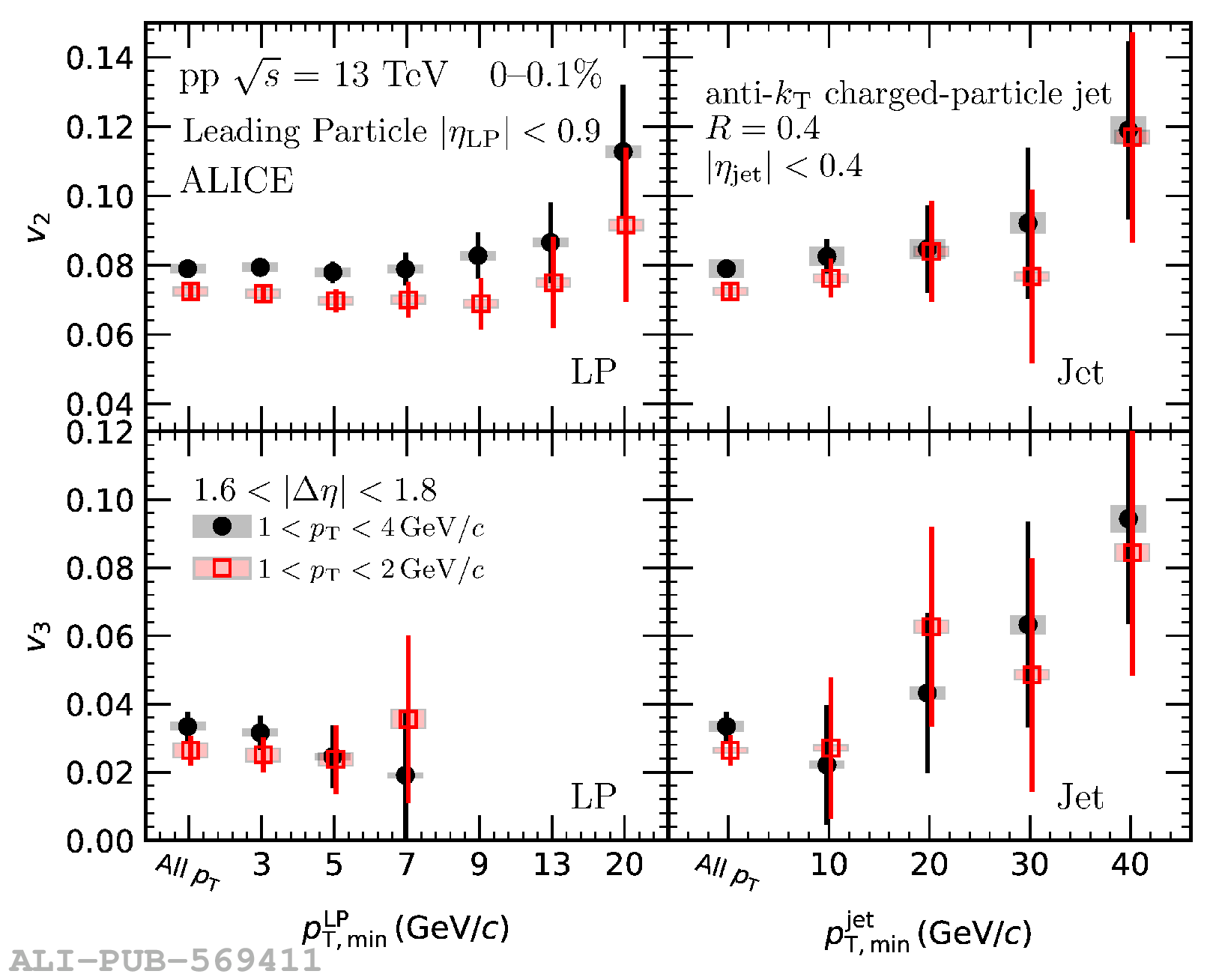# Figure 6

 The magnitudes of $v_2$ (top) and $v_3$ (bottom) as a function of $\it{p}^{\rm{LP}}_{\rm{T,min}}$ (left) and $\it{p}^{\rm{jet}}_{\rm{T,min}}$ (right) for the high-multiplicity in pp collisions at $\sqrt{s}=13$ TeV. The measured $p_{\mathrm{T}}$ intervals are $1< p_{\mathrm{T}}< 2\,\mathrm{GeV}/c$ (in red) and $1< p_{\mathrm{T}}< 4\,\mathrm{GeV}/c$ (in black). The statistical errors and systematic uncertainties are shown as vertical bars and boxes, respectively.